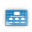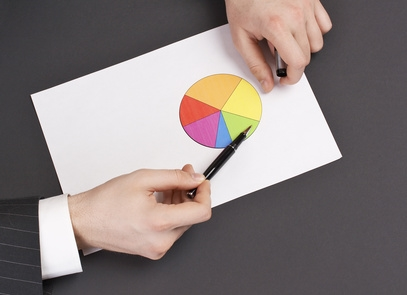This site uses cookies. By continuing to browse the ConceptDraw site you are agreeing to our Use of Site Cookies.# Basic Diagramming

This solution extends ConceptDraw DIAGRAM software with the specific tools you need to easily draw flowcharts, block diagrams, histograms, pie charts, divided bar diagrams, line graphs, circular arrows diagrams, Venn diagrams, bubble diagrams and concept maps, amongst others.

Benefits: Flowcharts, block diagrams, histograms, pie charts, divided bar diagrams, line graphs, circular arrows diagrams, Venn diagrams, bubble diagrams and concept maps, and others can be generated with ease.

Objective: Visually communicate business and personal ideas and concepts, general structures and activities.

Solution Purpose: Draw basic diagrams quickly and easily.

Intended For: Anyone who needs to draw a basic diagram.

Products and Methods: ConceptDraw DIAGRAM is a powerful intelligent vector graphics engine that can be used for basic diagramming.## Solution Reference

##### Toolbox 1. Basic diagrams

Draw flowcharts, block diagrams, histograms, pie charts, divided bar diagrams, line graphs, circular arrows diagrams, Venn diagrams, bubble diagrams, and concept maps for the visualizing of business information.

 Tasks Actions Products Flowchart In ConceptDraw DIAGRAM, use the template “Basic Flowchart” Basic Flowchart.cdt PRO 2D Block Diagram In ConceptDraw DIAGRAM, use the template “Blocks 2D” Blocks 2D.cdt PRO 3D Block Diagram In ConceptDraw DIAGRAM, use the template “Blocks 3D” Blocks 3D.cdt PRO Block Diagram with Perspective In ConceptDraw DIAGRAM, use the template “Blocks with Perspective” Blocks with Perspective.cdt PRO Histograms In ConceptDraw DIAGRAM, use the shape library “Histograms” Histograms.cdl PRO Pie Charts In ConceptDraw DIAGRAM, use the shape library “Pie Chart” Pie Charts.cdl PRO Divided Bar Charts In ConceptDraw DIAGRAM, use the shapes “Divided bar 1” and “Divided bar 2” from the library “Charts” Charts.cdl PRO Line Graphs In ConceptDraw DIAGRAM, use the shapes “Line Graph (Text ? Height)” and “Line Graph (Height ? Text)” from the library “Charts” Charts.cdl PRO Circular Arrows Diagram In ConceptDraw DIAGRAM, use the shape “Circular arrows” from the library “Marketing Diagrams” Marketing Diagrams.cdl PRO Venn Diagram In ConceptDraw DIAGRAM, use the template “Venn Diagram” Venn Diagram.cdt PRO Bubble Diagram In ConceptDraw DIAGRAM, use the template “Bubble Diagram” Bubble Diagram.cdt PRO Concept Map In ConceptDraw DIAGRAM, use the template “Concept Map” Concept Map.cdt PRO